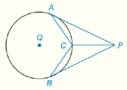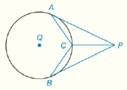Chapter 6.CR, Problem 29CRElementary Geometry For College St...

7th Edition
Alexander + 2 others
ISBN: 9781337614085

Solutions

Chapter
SectionElementary Geometry For College St...

7th Edition
Alexander + 2 others
ISBN: 9781337614085
Textbook Problem

In Review Exercises 27 to 29, give a proof for each statement. G i v e n :                 A P -   a n d   B P -   a r e   t a n g e n t   t o   ⊙ Q   a t   A   a n d   B                                                               C   i s   t h e   m i d p o i n t   o f   A B ^             P r o v e :                     P C →   b i s e c t s   ∠ A P BTo determine

To Prove: PC bisects APB, if AP- and BP- are tangent to Q at A and B. C is the midpoint of AB^.Explanation

Concept:

Theorem 6.1.3:

In a circle (or in congruent circles), congruent minor arcs have congruent central angles.

Theorem 6.1.3:

In a circle (or in congruent circles), congruent central angles have congruent minor arcs.

Theorem 6.1.5:

In a circle (or in congruent circles), congruent chords have congruent minor (major) arcs.

Theorem 6.1.6:

In a circle (or in congruent circles), congruent arcs have congruent chords.

Theorem 6.1.7:

Chords that are at the same distance from the center of a circle are congruent.

Theorem 6.1.8:

Congruent chords are located at the same distance from the center of a circle.

Theorem 6.2.4:

The measure of an angle formed by a tangent and a chord drawn to the point of tangency is one-half the measure of the intercepted arc.

Theorem 6.3.4:

The tangent segments to a circle from an external point are congruent.

Calculation:

Given AP- and BP- are tangent to Q at A and B. C is the midpoint of AB^.

By Theorem 6.3.4:

The tangent segments to a circle from an external point are congruent.

So, AP=BP

Since, C is the midpoint of AB^.

So, AC^=CB^

By, Theorem 6.1.6:

In a circle (or in congruent circles), congruent arcs have congruent chords.

Because congruent chords, AC=CB.

Theorem 6.1.3:

In a circle (or in congruent circles), congruent minor arcs have congruent central angles.

Since, AC^=CB^ then AQC=BQC

By Theorem 6

Still sussing out bartleby?

Check out a sample textbook solution.

See a sample solution

The Solution to Your Study Problems

Bartleby provides explanations to thousands of textbook problems written by our experts, many with advanced degrees!

Get Started

Find each limit. a. limx2| x2 |x2 b. limx2+| x2 |x2

Calculus: An Applied Approach (MindTap Course List)

Simplify: 1240

Elementary Technical Mathematics

Express the function in the form f g h. 51. S(t) = sin2(cos t)

Single Variable Calculus: Early Transcendentals, Volume I

The polar form for the graph at the right is:

Study Guide for Stewart's Multivariable Calculus, 8th

True or False: x2x24dx should be evaluated using integration by parts.

Study Guide for Stewart's Single Variable Calculus: Early Transcendentals, 8th